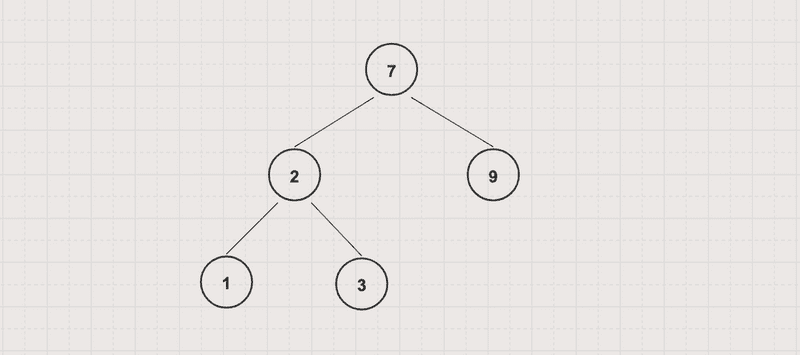# HelloKoding

Practical coding guides

# Height of a Binary Tree

In this article, you will learn to find height of a binary tree

The height of a binary tree is a number of edges between the tree’s root and its furthest leaf. For example, the height of the following binary tree is 2A tree consisting of only a single node has a height of 0

## Problem

Given a binary tree

Write an algorithm to find its height or maximum depth

## Approach

Using recursive algorithm, `height(root node) = max (height(left subtree of root node), height(right subtree of root node)) + 1`

BinaryTreeHeight.java

``````package com.hellokoding.algorithm;

public class BinaryTreeHeight extends BSTByLinkedList {
public int height(Node node) {
if (node == null) return -1;

return Math.max(height(node.left), height(node.right)) + 1;
}

public static void main(String[] args) {
BinaryTreeHeight tree = new BinaryTreeHeight();
tree.insert(7);
tree.insert(2);
tree.insert(3);
tree.insert(1);
tree.insert(9);

tree.inTraversal(tree.root);
System.out.println(tree.height(tree.root));
}
}
``````

`BSTByLinkedList` is defined in Binary Search Tree Data Structure